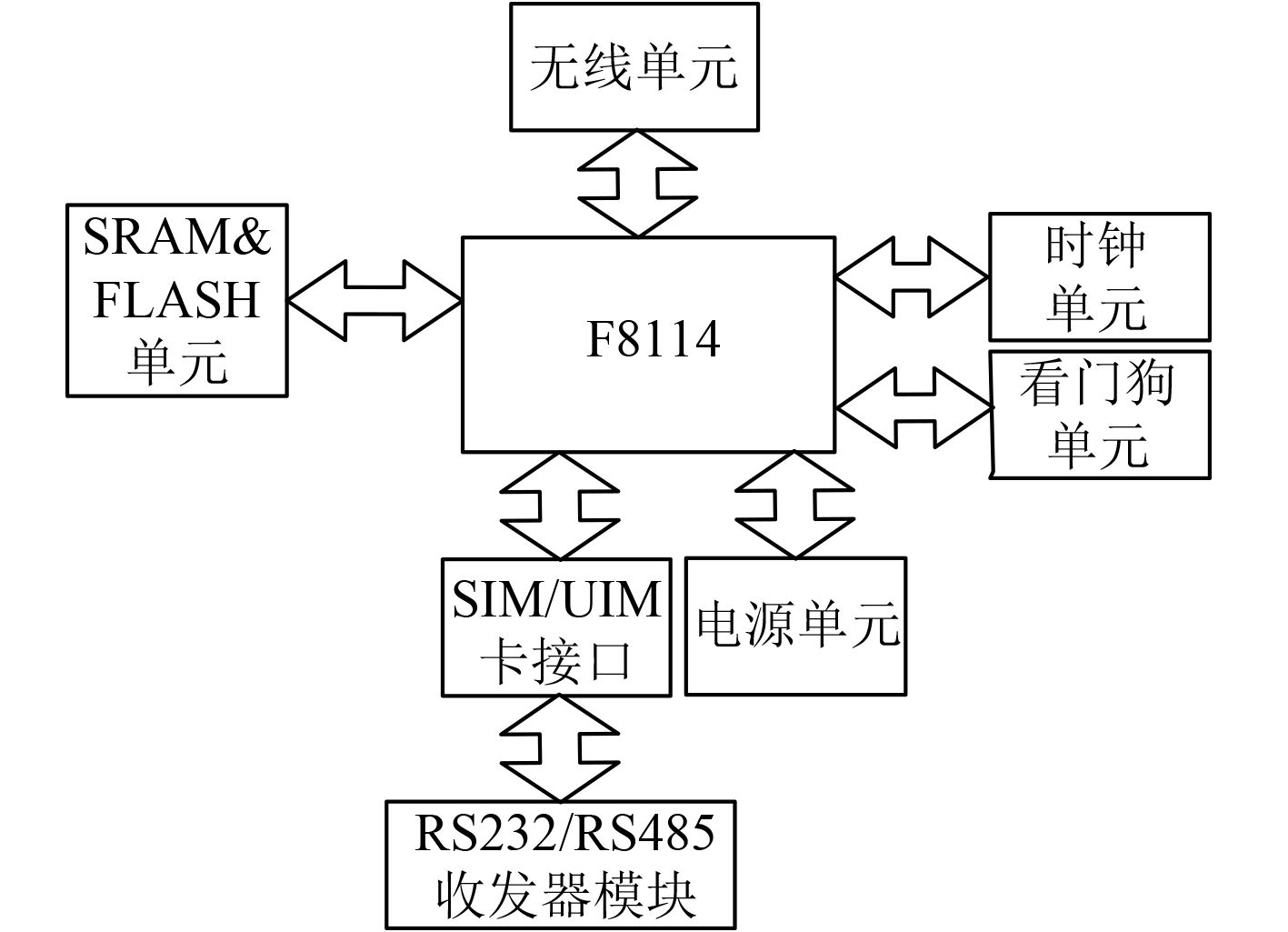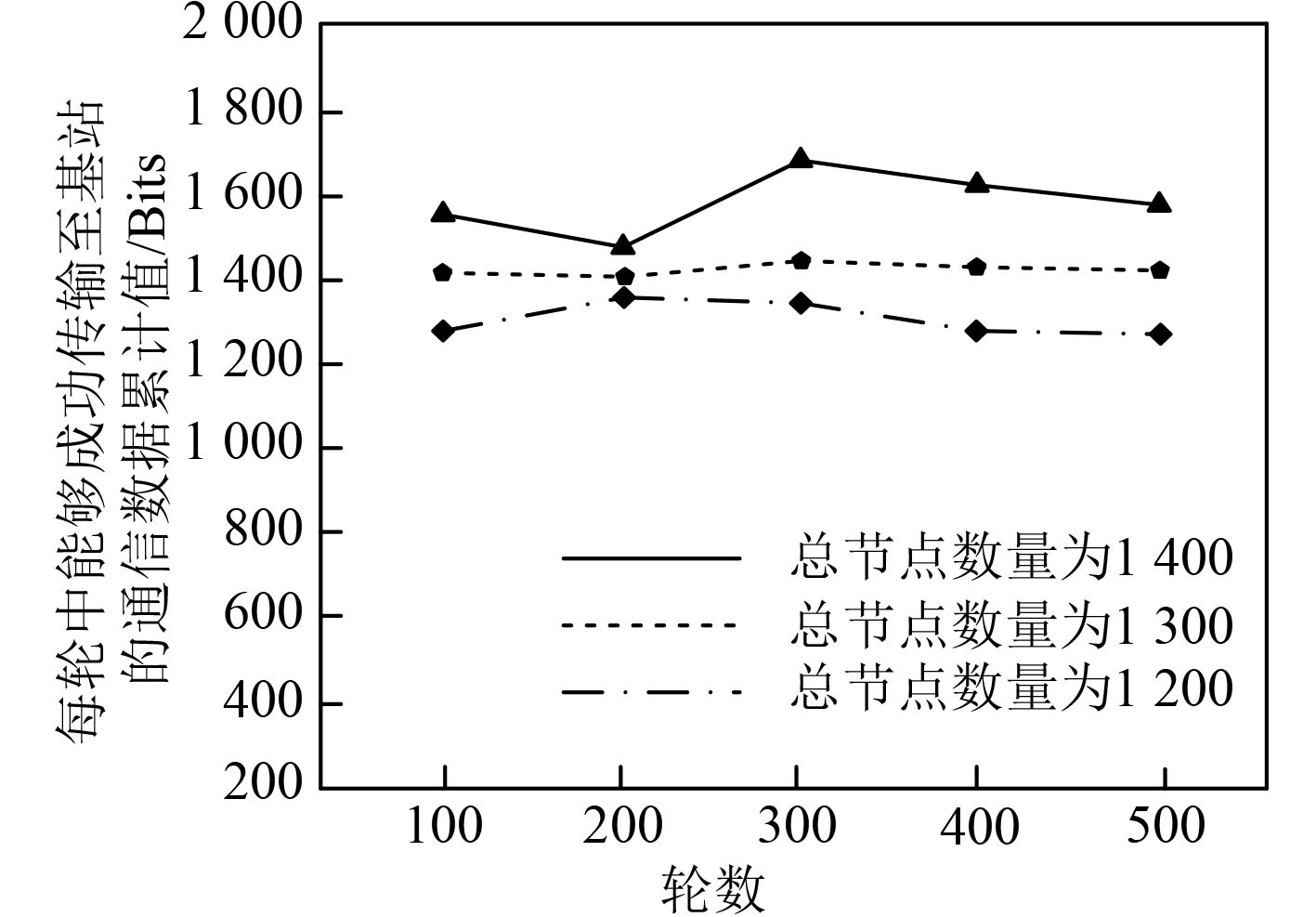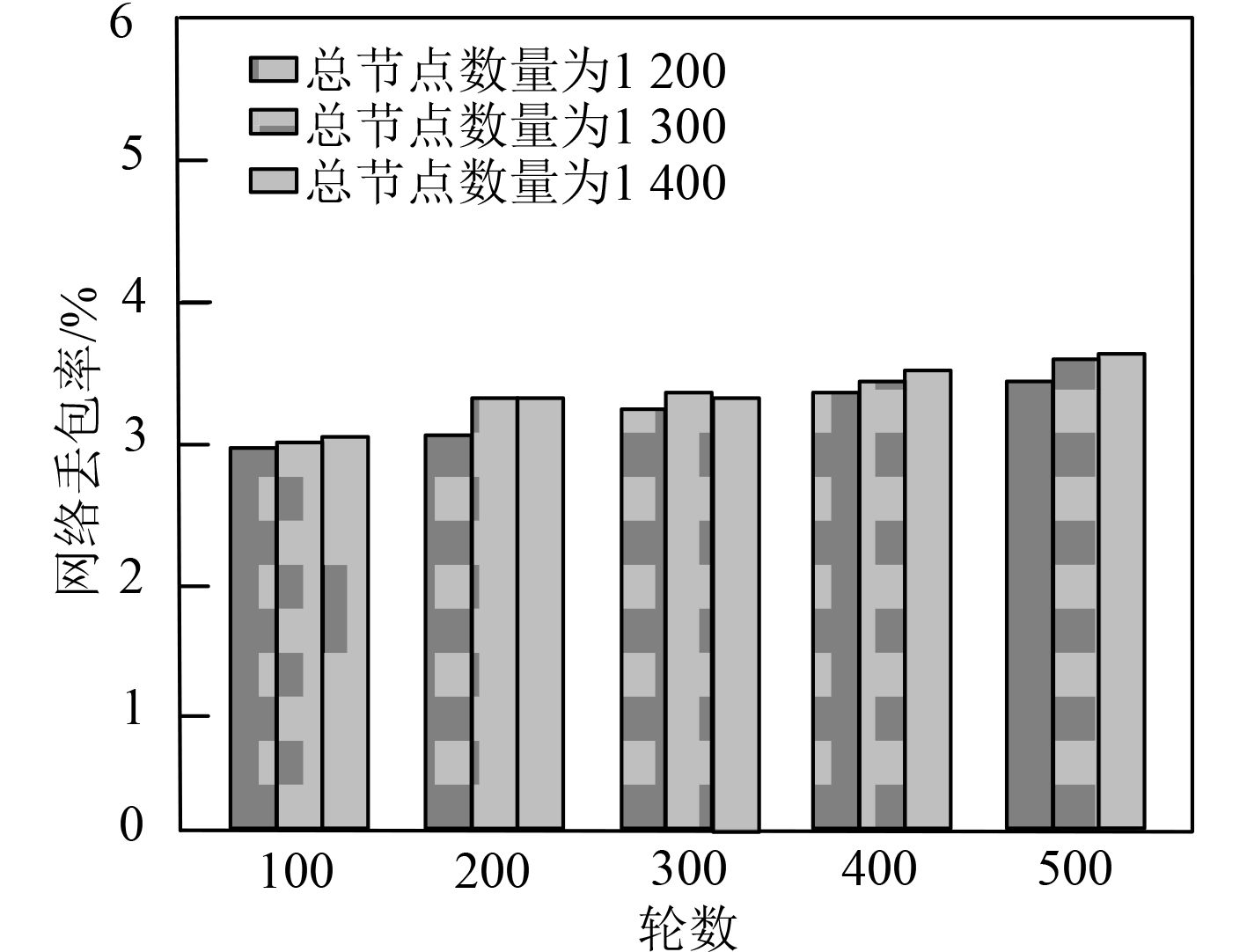﻿ 小波支持向量机船舶物联网监测拓扑结构设计
 舰船科学技术2022, Vol. 44Issue (7): 170-173    DOI: 10.3404/j.issn.1672-7649.2022.07.035PDF

1. 开封大学 信息工程学院, 河南 开封 475004;
2. 开封大学 人事处, 河南 开封 475004

Topological structure design of ship IoT monitoring based on wavelet support vector machine
LI Jing1, WEI Zhi-peng2
1. School of Information Engineering, Kaifeng University, Kaifeng 450046, China;
2. Personnel Department of Kaifeng University, Kaifeng 450046, China
Abstract: In the design of ship IoT monitoring topology, due to the large number of monitoring directions resulting in greater communication energy consumption and higher network packet loss rate, a ship IoT monitoring topology based on wavelet support vector machine was designed. Two data fusion algorithms are used to help this topology realize the ship monitoring function. Among them, for the data fusion of homogeneous sensors, the processing algorithm used is the first-level data fusion algorithm, and the data fusion between non-homogeneous sensors is realized through the second-level data fusion algorithm. Design a switch quantity prediction model based on wavelet support vector machine, determine the number of switches needed in the IoT monitoring topology, and complete the topology design. The communication performance of the designed topology is tested on a ship. The test results show that the throughput of the structure is high, the communication energy consumption is low, the average energy consumption of each node can be as low as 2.05 mJ, and the network packet loss rate is also low. It is proved that the designed structure has superior communication performance.
Key words: wavelet support vector machine     ship IoT monitoring     distribution mechanism     topology structure
0 引　言

1 小波支持向量机的船舶物联网监测拓扑结构 1.1 物联网监测拓扑结构设计

ZigBee模块是物联网监测拓扑结构中的基础模块，负责进行设备数据的采集。选择的ZigBee网关节点为F8914，能够与网关进行通信。在模块中布设16个信道，地址分配采用的是分配式机制，根据网络的最大深度、各父节点对应的子节点个数及其中的路由器节点个数对各节点的地址进行分配。分配后每个节点的物理地址唯一，网络地址均为16位图 1 网关节点单元的结构设计 Fig. 1 Structural design of gateway node unit

1.2 拓扑结构监测机能实现

1）将同质传感器的数量设为 $n$ ，对于第 $i$ 个传感器，将其测量值设为xi

2）对同质传感器测量得到的环境特征参数之间存在的相对偏差进行计算；

3）根据计算结果对置信距离矩阵 ${\boldsymbol{B}}$ 进行构建：

 ${\boldsymbol{B}} = \left[ {\begin{array}{*{20}{c}} {{B_{11}}}&{{B_{12}}}& \cdots &{{B_{1n}}} \\ {{B_{21}}}&{{B_{22}}}& \cdots &{{B_{2n}}} \\ \vdots & \vdots & \ddots & \vdots \\ {{B_{m1}}}&{{B_{m2}}}& \cdots &{{B_{mn}}} \end{array}} \right] 。$ (1)

4）对传感器数量中的被信任比例进行计算。以信任程度上限为依据对被信任比例小于0.5的传感器数据进行排除。其中信任程度上限用 $k$ 来表示，该值为传感器精度的2倍。对被排除传感器的数量进行统计，并用 $t$ 来表示。

5）对保留的同质传感器测量得到的环境特征参数进行计算，并求取其平均值，具体为：

 $\bar x = \frac{1}{{n - t}}\sum\limits_t^n {{x_i}} ，$ (2)

6）对 $\bar x$ 与同质传感器测量所获数据间的海明距离进行计算，具体为：

 ${d_i} = {\left| {{x_i} - \bar x} \right|^2} 。$ (3)

7）对 $\bar x$ 与同质传感器测量所获数据间的模糊距离贴近度进行计算，具体为：

 ${r_i} = \frac{1}{{n - t}}\left( {1 + {{\left( {{d_i}} \right)}^\varepsilon }} \right) 。$ (4)

8）对各同质传感器对应的权重值进行计算，即

 ${\omega _i} = \frac{{{r_i}}}{{\displaystyle\sum\limits_{i = 1}^{n - t} {{r_i}^2} }} 。$ (5)

9）对环境特征参数的对应一级融合值进行计算，公式如下：

 $X' = \sum\limits_{i = 1}^{n - t} {{\omega _i}{x_i}^2}。$ (6)

1）对因素集 $Y$ 进行确定，具体为：

 $Y = \left\{ {{y_1},{y_2},...,{y_n}} \right\}，$ (7)

2）对评语集进行确定：

 $C{\text{ = }}\left( {{c_1},{c_2},...,{c_m}} \right)，$ (8)

3）实施单因素评判，获取评判矩阵 ${\boldsymbol{A}}$

①对因素集 $Y$ 中的各因素实施评判，获取评语集 $E$ 到因素集 $F$ 的模糊映射 $p$ ，具体为：

 $p：F\to P\left(E\right)，$ (9)

②根据模糊映射对评语集到因素集之间的模糊关系进行诱导，获取模糊矩阵，具体为：

 ${\boldsymbol{V}} = \left[ \begin{gathered} {v_{11}}\;\;{v_{12\;\;\;}}{v_{13}} \hfill \\ {v_{21}}\;\;{v_{22\;\;\;}}{v_{23}} \hfill \\ {v_{31}}\;\;{v_{32\;\;\;}}{v_{33}} \hfill \\ {v_{41}}\;\;{v_{42\;\;\;}}{v_{43}} \hfill \\ {v_{51}}\;\;{v_{52\;\;\;}}{v_{53}} \hfill \\ \end{gathered} \right] 。$ (10)

1.3 确定节点数量与交换机数量

 $N = \left[ {\frac{{\sqrt {\dfrac{{\text{π}} }{{3S}}} }}{{2{R_C}}}{\text{ + }}\frac{1}{2}} \right] 。$ (11)

 ${N_{key}} = 3N\left( {N + 1} \right) - 1。$ (12)

 $f\left( x \right) = {\sum\limits_{i = 1}^{n - m} {0.01\left( {{a_1} - {a_2}} \right)} ^5}k\left( {x,{x_i}} \right) - 0.03b \cdot S 。$ (13)

2 性能测试 2.1 实验环境

2.2 性能测试与结果分析表 1 通信能耗测试结果 Tab.1 Test results of communication energy consumption图 2 网络吞吐量测试结果 Fig. 2 Network throughput test results图 3 网络丢包率测试结果 Fig. 3 Network packet loss rate test results
3 结　语

  沈艺敏. 基于物联网的温室农业种植环境监控系统研究[J]. 农机化研究, 2022, 44(6): 209-213. DOI:10.3969/j.issn.1003-188X.2022.06.036  侯加林, 蒲文洋, 李天华, 等. 基于UWB与物联网的移动式温室环境监测系统设计与实现[J]. 农业工程学报, 2020, 36(23): 229-240. DOI:10.11975/j.issn.1002-6819.2020.23.027  段磊, 廖根根, 彭静, 等. 全站仪自动监测系统的构建方法及其在地铁监测中的应用[J]. 工程技术研究, 2021, 6(3): 1-3. DOI:10.3969/j.issn.1671-3818.2021.03.001  袁伟杰, 李双洋, 种若汐, 等. 面向6G物联网的分布式译码技术[J]. 电子与信息学报, 2021, 43(1): 21-27. DOI:10.11999/JEIT200343  涂经科, 梁俊斌, 蒋婵, 等. 大规模无环有向管网中移动物联网监测进展[J]. 计算机工程与应用, 2020, 56(17): 1-11.  陶志刚, 任树林, 庞仕辉, 等. 无煤柱自成巷大数据物联网监测系统及工程应用[J]. 中国矿业大学学报, 2021, 50(5): 983-991.  张孟伦, 张希, 牛鹏飞. 面向物联网监测节点的集成式微流控传感芯片[J]. 传感技术学报, 2021, 34(2): 157-161. DOI:10.3969/j.issn.1004-1699.2021.02.003  崔立群, 伍军. 信息中心物联网节点状态监测技术研究[J]. 中国工程科学, 2020, 22(6): 121-127.  胡飞虎, 郭建文, 吴阿丹, 等. 基于Python的生态监测物联网数据自动采汇中间件应用研究[J]. 遥感技术与应用, 2020, 35(2): 478-483.